# Elements

## Solids

The 8-node solid element by default uses one point integration plus viscous hourglass control. Fully integrated brick elements are also available – see `*SECTION_SOLID` (Material Card 1, Column 80 for `IARB=0`, Property Set Card 1, Column 25 for `IARB=1`) and Section 3.3 of the Theory Manual- ; these perform better where element distortions are large but are about four times more costly. No hourglass control is needed as there are no zero-energy modes. Wedges and tetrahedra are simply degenerate bricks (i.e some of the nodes are repeated); they cause problems in some situations and are best avoided.

## Shells

All shell elements include membrane, bending and shear deformation. The default Belytschko-Tsay formulation is the most economical and should be used unless features particular to other formulations are required e.g.:

Hughes Liu: can o set the mid-plane of the element away from the nodes.

S/R co-rotational Hughes-Liu: fully integrated, so hourglass deformations do not occur (but much more costly).

Belytschko-Tsay membrane: (and fully integrated membrane): appropriate for fabrics etc. where bending stiffness is negligible.

As degenerate quadrilateral shell elements are prone to lock under transverse shear, triangular shell elements have now been implemented, based on work by Belytschko and co-workers. Triangular shells can be mixed with quadrilateral shells within the same material property set, provided that the element sorting flag ITRIST on `*CONTROL_SHELL` (Control Card 12, Column 20) is set to 1.

Three-dimensional plane stress constitutive subroutines are implemented for the shell elements which update the stress tensor such that the stress component normal to the shell mid surface is zero. The integration points are stacked vertically at the centroid of the element, as shown in Figure ??.

Through-thickness directions at each node are initially normal to the element surface but rotate with the nodes. Strains are linear through the thickness. Two integration

Solid Elements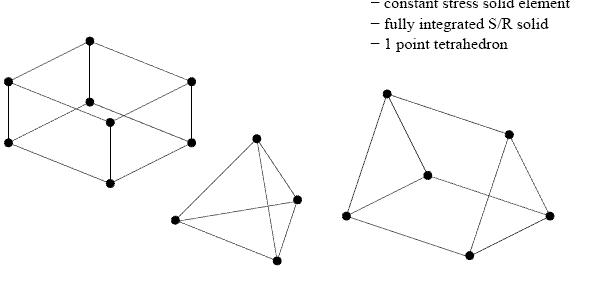Shell Elements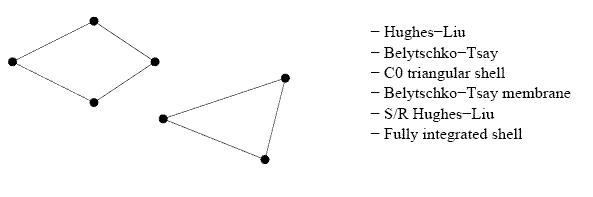Beam and Truss Elements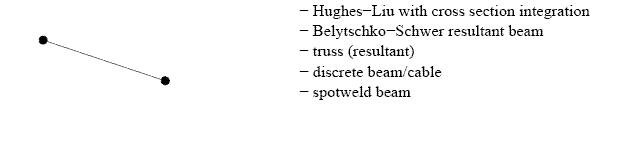Discrete Elements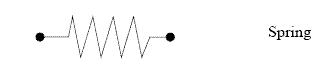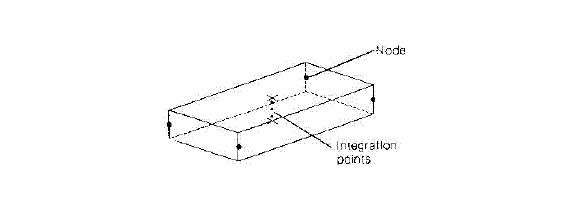Figure 10.01: Integration Points

points are su cient for linear elastic material, while more points are required for nonlinear material. Stress output gives stresses at the outermost integration points, not at the surfaces (despite the nomenclature of post-processors, which refer to top and bottom surfaces), so care is needed in interpretation of results. For elastic materials, stresses can be extrapolated to the surfaces. For nonlinear materials the usual policy is to choose four or five integration points through the thickness and to ignore the error (i.e. the di erence in stress between the surface and the outermost integration point). The location of the outermost integration points for Gauss quadrature are given in the following table: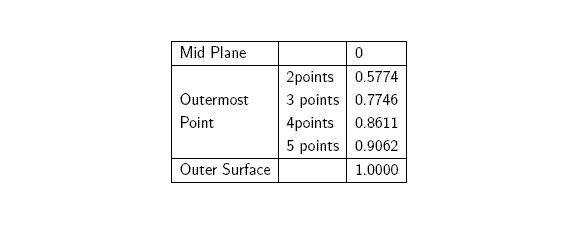## Newton-Cotes Formulas

In the Newton-Cotes method r pairs of weights wi and regularly spaced coordinates ξi integrate a polynomal of degree r – 1 exactly.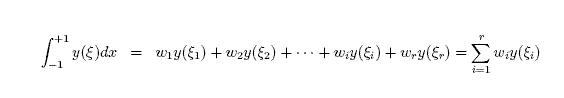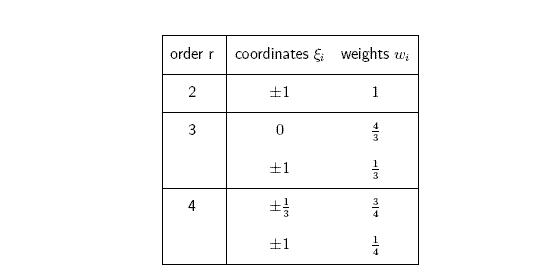Trapezoidal Rule (r=2, domain divided into n subdomains)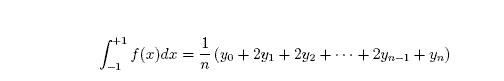Simpson Rule (r=3, domain divided into n subdomains)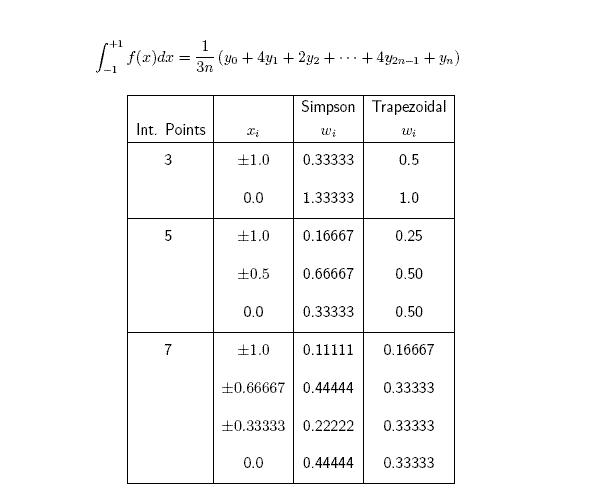## Gauss Integration

In the Gauss method r pairs of weights wi and coordinates ξi integrate a polynomal of degree `m <= 2r - 1` exactly.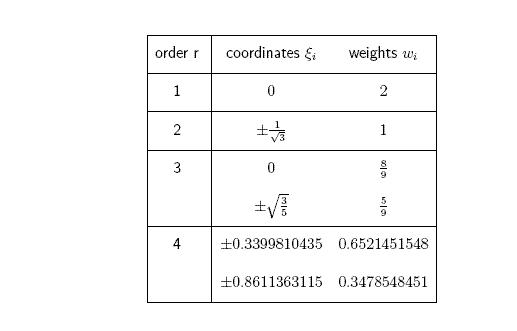## Gauss Lobatto Integration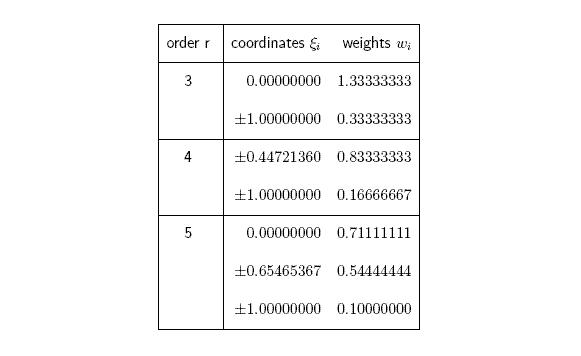Figure 10.2 shows the displacement of a cantilever beam subjected to a load at the free end.

This figure shows the poor behaviour of the Trapezoidal integration rule (order r=2) using up to 19 subdomains (20 integration points) compared with other Newton-Cotes formulas without using subdomains. Using order r=3 (Simpson points) already gives the correct result.

Figure 10.2: Poor behaviour of trapezoidal rule

## Location of Integration Points Through Thickness

In LS-DYNA the location of integration points through thickness of shell elements for LS-POST database depends on

• database (d3plot or ASCII database elout)
• number of shell integration points written to the d3plot database, MAXINT on `*DATABASE_EXTENT_BINARY`, (Control Card 21, Column 20)
• quadrature rule (Gauss, trapezoidal, user defined)

Assume a shell element with five through thickness integration points. Then the location of these integration points for LS-POST database is as shown in figures 10.3 and 10.4.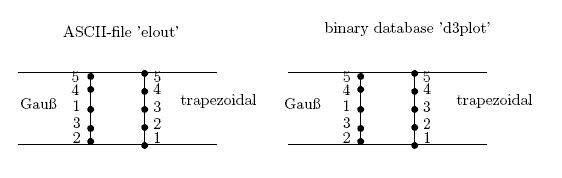Figure 10.3: Location of integration points for `NIP=5`, `MAXINT=5`

```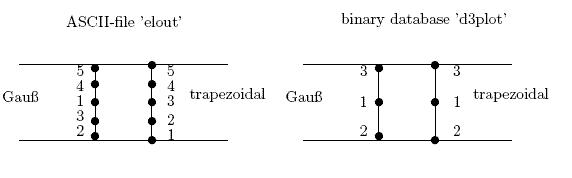```

Figure 10.4: Location of integration points for `NIP=5`, `MAXINT=3`